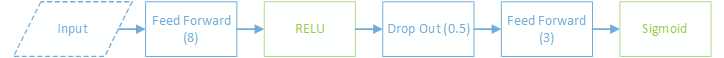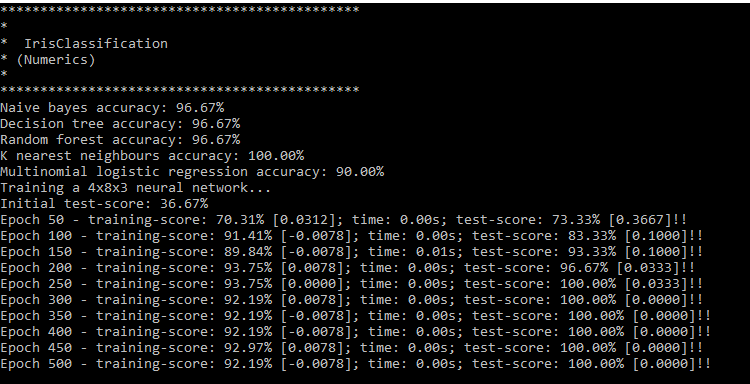# Training Naive Bayes, Decision Tree, Random Forest, KNN, Multinomial Logistic Regression and Neural Network classifiers on the Iris data-set.

## Something to Classify

The Iris data-set is a simple, 150 row flower classification data set.

```var reader = GetStreamReader(context, "iris.csv", "https://archive.ics.uci.edu/ml/machine-learning-databases/iris/iris.data");
try
{
using var table = context.ParseCsv(reader, false);
table.SetTargetColumn(4);
using var numericTable = table.ConvertTable(
ColumnConversionType.ToNumeric,
ColumnConversionType.ToNumeric,
ColumnConversionType.ToNumeric,
ColumnConversionType.ToNumeric);
using var normalized = numericTable.Normalize(NormalizationType.FeatureScale);
return new IrisTrainer(normalized.AsRowOriented(), table.Column(4).ToArray<string>());
}
finally
{
}```

Bright Wire includes a tabular data table that can be created directly from a StreamReader (assuming the stream reader contains valid CSV).

The Iris dataset contains five columns of data. The first four are sepal and petal measurements and the last column is the Iris class (Iris Setosa, Iris Versicolour or Iris Virginica).

We'll use all four numeric measurements as features and the class label as the classification target.

## Evaluating some Classifiers

Now that we have our data, let's do some machine learning! We'll start with a Naive Bayes classifier.

We'll train the classifier on the training data and evaluate it using the test data.

```var ret = Training.TrainNaiveBayes();
var results = Test.Classify(classifier).ToList();
var score = results
.Average(d => d.Row.GetTyped<string>(TargetColumn) == d.Classification.OrderByDescending(c => c.Weight).First().Label ? 1.0 : 0.0);
Console.WriteLine(\$"{type} accuracy: {score:P}");```

The classification accuracy is around 97% from one of the simplest machine learning classifiers. Not bad. Let's move on to a decision tree classifier.

```var ret = Training.TrainDecisionTree();
if (writeResults)
WriteResults("Decision tree", ret.CreateClassifier());```

It turns out that both the Naive Bayes classifier and the Decision Tree get exactly the same accuracy - around 97%. The exact accuracy depends on which samples are split into in the training and test data sets after the shuffle.

It might be tempting to train on the entire data set which would easily take the accuracy to 100% rather than using the training and test splits. But That Would Be Bad (our goal is not to teach the computer the learning data, but rather to generalise on data it hasn't seen yet)

Let's try a random forest...

```var ret = Training.TrainRandomForest(numTrees, baggedRowCount);
if(writeResults)
WriteResults("Random forest", ret.CreateClassifier());```

The random forest accuracy is the same - around 97%. Is there a pattern here somewhere? ; )

Naive Bayes and Decision Trees aren't linear algebra based machine learning algorithms (they don't use vectors or matrices). To use other algorithms we will need to create a linear algebra provider.

Bright Wire supports CPU based linear algebra. If you have a NVIDIA GPU you can also add GPU based linear algebra with the CUDA add on. Note that the standard version can take advantage of CPU based optimisation libraries such as the Math.Net Numerics MKL library.

However this is such a small data set that the CPU based linear algebra will be fine.

## K Nearest Neighbours

K Nearest Neighbours compares each test example with the entire training set. Let's see how it goes...

```var ret = Training.TrainKNearestNeighbours();
if (writeResults)
WriteResults("K nearest neighbours", ret.CreateClassifier(Table.Context.LinearAlgebraProvider, k));```

The accuracy is the same - around 97%.

## Multinomial Logistic Regression

Multinomial Logistic Regression is a similar story getting the same 97% accuracy.

```var ret = Training.TrainMultinomialLogisticRegression(iterations, trainingRate, lambda);
if (writeResults)
WriteResults("Multinomial logistic regression", ret.CreateClassifier(Table.Context.LinearAlgebraProvider));```

## Neural Network

To train a neural network we'll first need to convert our data table into vectors. The default conversion is to convert continuous features (such as the first four columns of our data) into a Single (float) and to one-hot encode categorical features (such as the class label) into multiple values per category (in this case into 001, 010 or 100 depending on the class label).

The converter will convert each row in the table into two vectors, one for the network input and the other for the expected output - in this case the first vector will be length 4 (for the four features) and the second vector will be length 3 (for the three possible class labels).

```// convert the data tables into linear algebra friendly training data providers
var trainingData = lap.NN.CreateTrainingDataProvider(split.Training);
var testData = lap.NN.CreateTrainingDataProvider(split.Test);```

A basic neural network design is to have two feed forward layers separated by a drop out layer. The drop out layer helps to regularise the network.

In this case our first layer has 8 neurons and RELU activation and the final (output) layer has two neurons and sigmoid activation.

The network is trained with rms prop gradient descent and a mini batch size of 8.```// create a neural network graph factory
var graph = Table.Context.CreateGraphFactory();

// the default data table -> vector conversion uses one hot encoding of the classification labels, so create a corresponding cost function
var errorMetric = graph.ErrorMetric.OneHotEncoding;

// create the property set (use rmsprop gradient descent optimisation)
graph.CurrentPropertySet
.Use(graph.RmsProp())
.Use(graph.GaussianWeightInitialisation(true, 0.1f, GaussianVarianceCalibration.SquareRoot2N));

// create the training and test data sources
var trainingData = graph.CreateDataSource(Training);
var testData = trainingData.CloneWith(Test);

// create a 4x8x3 neural network with sigmoid activations after each neural network
var engine = graph.CreateTrainingEngine(trainingData, trainingRate, batchSize, TrainingErrorCalculation.TrainingData);
graph.Connect(engine)

The output vector will correspond to the network's classification of the input vector (the categories are indexed by the index of the maximum output value in the output vector).

The one-hot error metric will check how well the maximum index is corresponding to the labelled training data and the network will adjust itself accordingly.

```// train the network
Console.WriteLine("Training a 4x8x3 neural network...");
engine.Train(numIterations, testData, errorMetric, null, 50);```

## Output## Summary

All classifiers did really well on this data set. But that's only because the data set is trivially easy to classify. With only 150 rows it's also very small. We can't say which classifier is "best" under these conditions.

The only valid conclusion is that Bright Wire makes it easy to try a suite of different classifiers in .NET ; )

## Complete Source Code

View the complete source on GitHub.